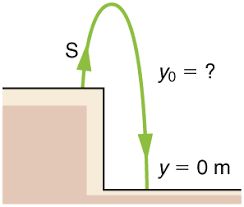## How to Calculate and Solve for Reaction: Lift Falls Freely | MotionThe image above represents reaction: lift falls freely.

To compute for reaction: lift falls freely, two essential parameters are needed and these parameters are mass (m) and acceleration due to gravity (g).

The formula for calculating reaction: lift falls freely:

R = mg

Where;

R = Reaction
m = Mass
g = acceleration due to gravity

Let’s solve an example;
Find the reaction when the mass is 30 and acceleration due to gravity is 9.8.

This implies that;

m = Mass = 30
g = acceleration due to gravity = 9.8

R = mg
R = 30 x 9.8
R = 294

Therefore, the reaction is 294 N.

Calculating the Mass when the Reaction and the Acceleration due to Gravity is Given.

m = R / g

Where;

m = Mass
R = Reaction
g = acceleration due to gravity

Let’s solve an example;
Find the mass when the reaction is 40 and the acceleration due to gravity is 9.8

This implies that;

R = Reaction = 40
g = acceleration due to gravity = 9.8

m = R / g
m = 40 / 9.8
m = 40 / 9.8
m = 4.08

Therefore, the mass is 4.08.